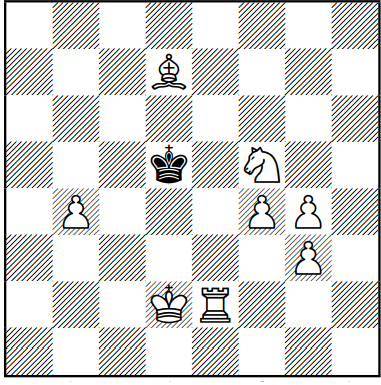# Chess Composition

In the position below, how can White give checkmate in two moves?The target square of the first move can be represented as a coordinate $(x,y)$, where the bottom left corner square is at $(1,1)$. Input the product of $x$ and $y$ as your answer.

×